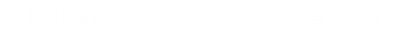# Calculating Invoices

As of Lawtrac 4.1.1, invoices will be calculated using the "Banker's Rounding System". This page details how to calculate invoices using this system. Additionally, this page will provide a CFC template which holds all of the Banker's Rounding functions.

## Banker's Rounding Quick Guide:

• Round down if the thousandths digit <=4
• Round up if the thousandths digit >=6
• If the thousandths digit =5, round up if there are any non-zero digits beyond the thousandths digit
• If the thousandths digit = 5 and all other digits beyond thousandths are 0, round up or down to the nearest even number

## Banker's Rounding Examples

• 0.0150 = 0.02
• 0.0520 = 0.02
• 0.0251 = 0.03
• 0.0349 = 0.03
• 0.0350 = 0.04

## Invoice Calculation

Foreign Detailed Invoice Line Items
The Unit Cost value of a detailed foreign invoice can display up to 4 decimal digits.
For example, before the adjustment the unit cost would equal €1,000.0000. During the adjustment, the unit cost would equal €1,000.1234. After the adjustment, the unit cost would equal €1,000.1234.

The Summary Fees, Disbursements, Others, and Adjustments of a foreign summary invoice should always use banker's rounding and display up to 2 decimal digits.

Base Currency Detailed Invoice Line Item
The Unit Cost value of a base currency invoice will always display up to 2 decimal digits.
For example, before the adjustment the unit cost would equal \$300.00. During the adjustment, the unit cost would equal \$300.1234. After the adjustment, the unit cost would equal \$300.12.

The Summary Fees, Disbursements, Others, and Adjustments of a base currency summary invoice should always use banker's rounding and display up to 2 decimal digits.